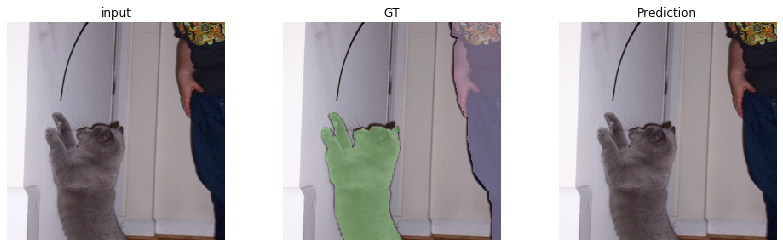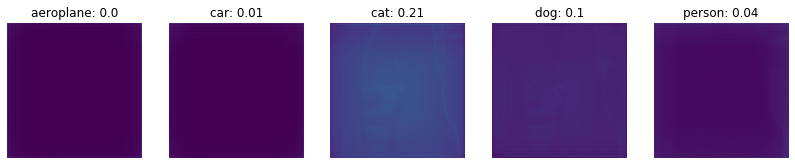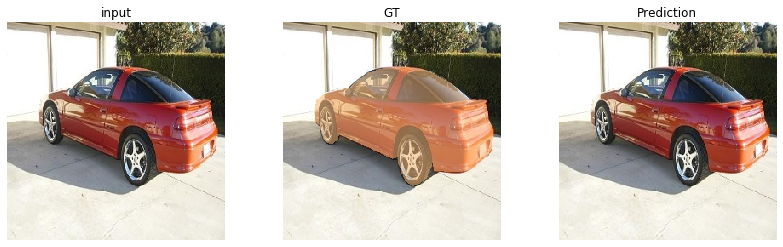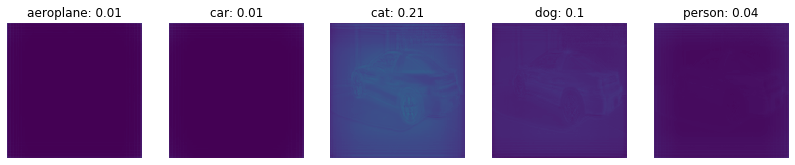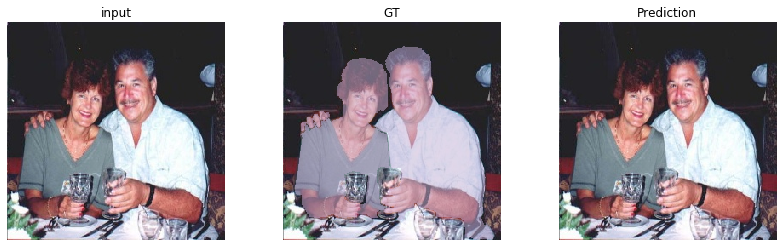# Transfer Learning Workshop 2¶

## Transfer Learning Across Tasks: From Classification To Semantic Segmentation¶

Welcome to day 2 of ComputeFest 2020! In this workshop, we will explore and play around with the common applications of transfer learning. Transfer learning can be used to improve performance on small datasets, across tasks, and even to recognize and properly process unseen examples.

The flow of the workshop is as follows:

1. The basics of transfer learning
2. Transfer Learning from classification to segmentation
3. Transfer learning through distillation

• Transfer learning is not only useful for simple classification task (e.g. Workshop 1).
• Pre-trained classification models can even transfer knowledge to other tasks like semantic segmentation.

## Goal¶

• Understand the task of sementic segmentation
• Get familiar with some segmentation networks
• Build your own segmentation network
• Improve your segmentation network by transfering knowledge from classification networks

## Structure¶

1. Introduction to Semantic Segmentation.

1.1 What is semantic segmentation?

1.2 Examples

1.3 Two common proposed networks: FCN and U-Net

2. Let’s dive into the code

2.1 Setup

2.2 Input pipeline

2.3 The segmentation network architecture

2.4 Training the network from scratch

2.5 Visualize results

3. Transfer Learning to the rescue: again?

3.1 Re-run training with pretrained encoder weights

3.2 Quantitative results

3.3 Qualitative results

3.4 Running on webcam images

3.5 Running on external images

# 1. Introduction to Semantic Segmentation.¶

## 1.1 What is semantic segmentation?¶

• Classification: assigning a single label to the entire picture.
• Semantic segmentation: assigning a semantically meaningful label to every pixel in the image.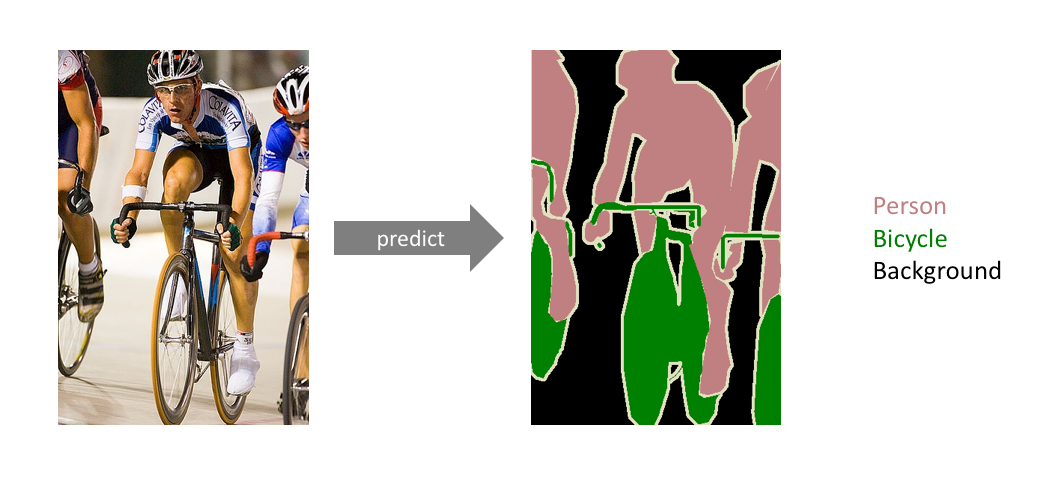## 1.2 Examples¶

• Autonomous vehicles
• Biomedical Imaging
• Aerial Surveying
• Geo Sensing, etc.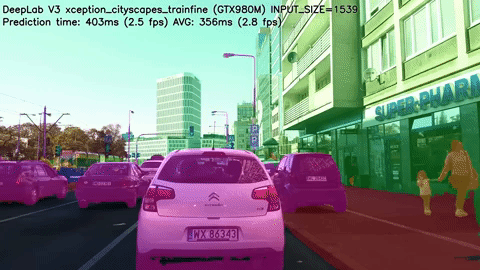## 1.3 Two common network architectures¶

Note that current state-of-the-art segmentation architectures tend to differ (sometimes quite significantly) from the architectures presented below, but for brevity and simplicity, we restrict it to these two.

• FCN: Fully-Convolutional Networks. (Paper)

• How can we transform a Classification Network to Segmentation Network?

Changing the fully connected layers into convolution layers enables a classification net to output a heatmap.• Adding layers and a spatial loss (between the output and the segmentation mask) produces an efficient framework for end-to-end dense prediction learning.
• The FCN can be seen as an encoder and decoder network:

• Encoder: Spatially downsample the input image to a smaller size (while gaining more channels) through a series of convolutions
• Decoder: The encoded output is then upsampled either through bilinear interpolation or a series of transposed convolutions to yield a high-resolution dense prediction.• U-Net: a symetric FCN architecture with skip connections. (Paper)

• U-Net consists of an almost symetric encoder and decoder.
• Most importantly it has skip connections from the output of convolution blocks to the corresponding input of the transposed-convolution block at the same level: these skip connections allow gradients to flow more effectively and provides information from multiple scales of the image.# 2. Let’s dive into the code:¶

## 2.1 Setup¶

In this section, we set up the working directory and color palettes for visualization.

Note that retrieving the dataset can take a few minutes.

In :
!git clone https://github.com/VincentCa/transfer_learning.git

import os
os.chdir("transfer_learning")
!ls

Cloning into 'transfer_learning'...
remote: Enumerating objects: 3, done.
remote: Counting objects: 100% (3/3), done.
remote: Compressing objects: 100% (3/3), done.
remote: Total 46001 (delta 0), reused 1 (delta 0), pack-reused 45998
Receiving objects: 100% (46001/46001), 2.49 GiB | 16.46 MiB/s, done.
Resolving deltas: 100% (11660/11660), done.
Checking out files: 100% (18226/18226), done.
deeplab_model.py  ImageSets   model_pretrained.h5  __pycache__
helpers.py	  JPEGImages  model_scratch.h5	   SegmentationClassSubset

In :
%load_ext autoreload
%tensorflow_version 2.x

import helpers
from helpers import *
%matplotlib inline
from matplotlib.colors import ListedColormap
from matplotlib import cm
from matplotlib import gridspec
from matplotlib import pyplot as plt
import numpy as np

cmap_ref = cm.get_cmap('tab20', 12)
cmap_seg = np.zeros((6, 4))
cmap_seg = [0.7, 0.7, 0.7, 0]
for i in range(1, 6):
cmap_seg[i] = cmap_ref(i)

cmap_seg = ListedColormap(cmap_seg)
print("\nClasses to detect, with corresponding colors:")
plt.imshow([[0, 1, 2, 3, 4, 5]], cmap=cmap_seg)
plt.xticks([0,1 ,2 ,3 ,4 ,5, 6], LABEL_NAMES[[0, 1, 7, 8, 12, 15]], rotation=45)

INPUT_SPATIAL = 224

TensorFlow 2.x selected.

Classes to detect, with corresponding colors: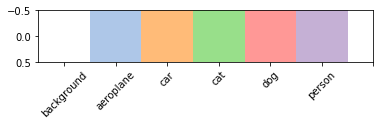In :
source_raw = 'JPEGImages'

with open('ImageSets/Segmentation/train.txt', 'r') as fp:
files_train = [line.rstrip() for line in fp.readlines()]

with open('ImageSets/Segmentation/val.txt', 'r') as fp:
files_val = [line.rstrip() for line in fp.readlines()]

# Filter down to the subset we are using.
files_train = [f for f in files_train if os.path.isfile(os.path.join('SegmentationClassSubset/' + f + '.npy'))]
files_val = [f for f in files_val if os.path.isfile(os.path.join('SegmentationClassSubset/' + f + '.npy'))]

# Split train-validation into 80:20 instead of the original split.
files_all = np.array(sorted(list(set(files_train).union(set(files_val)))))
index = int(len(files_all) * 0.8)
files_train = files_all[:index]
files_val = files_all[index:]
print(len(files_train), 'training', len(files_val), 'validation')
labels = ['background', 'aeroplane', 'car', 'cat', 'dog', 'person']

792 training 199 validation


## 2.2 Input pipeline¶

In this section, we define two generators to provide data to the network: one for training and one for validation data.

We then define the network architecture: we are using a Keras pre-defined version of MobileNet-v2 as encoder, and build a very simple and light-weight matching decoder on top. We also add skip-connections, similar to U-NET. We end up with an encoder-decoder architecture of matching input/output resolution.

Finally, we define a slightly modified cross-entropy loss that takes border/unlabeled pixels into account through masking.

In :
gen_train = CustomDataGenerator(source_raw=source_raw,
filenames=files_train.copy(),
batch_size=4,
target_height=INPUT_SPATIAL,
target_width=INPUT_SPATIAL)

gen_val = CustomDataGenerator(source_raw=source_raw,
filenames=files_val.copy(),
batch_size=4,
target_height=INPUT_SPATIAL,
target_width=INPUT_SPATIAL)


Here, we visualize an example pair produced by the generator. The generator marks border/unlabeled pixels as -1, background as 0 and specific classes as 1 and over.

In :
X, Y = gen_train
X = X
Y = Y

plt.figure(figsize=(9, 4))
plt.subplot(1, 2, 1)
plt.imshow(norm_vis(X, mode='rgb'))
plt.subplot(1, 2, 2)
plt.imshow(Y[:, :, 0])
plt.show()

print('X shape', X.shape, 'min-mean-max', X.min(), X.mean(), X.max())
print('Y shape', Y.shape, 'min-mean-max', Y.min(), Y.mean(), Y.max())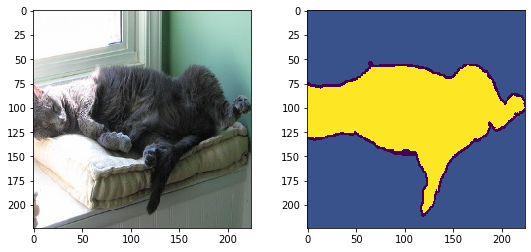X shape (224, 224, 3) min-mean-max -1.0 0.029298135390676255 1.0
Y shape (224, 224, 1) min-mean-max -1 0.8687420280612245 3


## 2.3 The segmentation network architecture¶

In :
K.clear_session()

def conv_block_simple(prev, num_filters, name):
return Conv2D(num_filters, activation='relu', kernel_size=(3, 3), padding='same', name=name + '_3x3')(prev)

selected_encoder = tf.keras.applications.mobilenet_v2.MobileNetV2(
input_shape=(INPUT_SPATIAL, INPUT_SPATIAL, 3),
include_top=False,
alpha=1.0,
weights='imagenet' if pretrained else None)
for l in selected_encoder.layers:
l.trainable = True
if verbose:
print(l.name, l.output.shape)

conv0 = selected_encoder.get_layer("expanded_conv_project").output # 112 x 112
conv1 = selected_encoder.get_layer("block_2_project").output # 56 x 56
conv2 = selected_encoder.get_layer("block_5_project").output # 28 x 28
conv3 = selected_encoder.get_layer("block_12_project").output # 14 x 14

up6 = selected_encoder.output
conv7 = up6

up8 = concatenate([UpSampling2D()(conv7), conv3], axis=-1)
conv8 = conv_block_simple(up8, 128, "conv8_1")

up9 = concatenate([UpSampling2D()(conv8), conv2], axis=-1)
conv9 = conv_block_simple(up9, 64, "conv9_1")

up10 = concatenate([UpSampling2D()(conv9), conv1], axis=-1)
conv10 = conv_block_simple(up10, 32, "conv10_1")

up11 = concatenate([UpSampling2D()(conv10), conv0], axis=-1)
conv11 = conv_block_simple(up11, 32, "conv11_1")

up12 = UpSampling2D()(conv11)
conv12 = conv_block_simple(up12, 32, "conv12_1")

x = Conv2D(N_CLASSES, (1, 1), activation=None, name="prediction")(conv12)

x = tf.keras.layers.Activation("softmax")(x)

model = tf.keras.Model(selected_encoder.input, [x] * n_outputs)
if verbose:
model.summary()
return model

In :
def masked_loss(y_true, y_pred):
"""Defines a masked loss that ignores border/unlabeled pixels (represented as -1).

Args:
y_true: Ground truth tensor of shape [B, H, W, 1].
y_pred: Prediction tensor of shape [B, H, W, N_CLASSES].
"""
gt_validity_mask = tf.cast(tf.greater_equal(y_true[:, :, :, 0], 0), dtype=tf.float32) # [B, H, W]

# The sparse categorical crossentropy loss expects labels >= 0.
# We just transform -1 into any valid class label, it will then be masked anyways.
y_true = K.abs(y_true)
raw_loss = tf.keras.losses.sparse_categorical_crossentropy(y_true, y_pred)  # [B, H, W].



## 2.4 Training the network from scratch¶

Given the very small dataset, it is hard to train a large architecture such as MobileNet-v2 and accompanying decoder robustly. As we see below, training from scratch leads to very strong overfitting, and is not successful.

In :
model_scratch = get_fcn(pretrained=False, verbose=False)

print('total number of model parameters:', model_scratch.count_params())

total number of model parameters: 3984166


We now run training. Depending on the GPU assigned, this should take about 4 minutes.

In :
history_scratch = model_scratch.fit(gen_train, epochs=20, verbose=1, validation_data=gen_val)


If you encounter any problems with above training cell, you can uncomment the cell below to load weights that we pre-trained using the same code.

In :
# Uncomment to restore pre-trained weights instead:


## 2.5 Visualize results¶

In :
def show_examples(model, deeplab=False):
for i in [2, 13, 10, 9]:
img = cv2.imread(os.path.join(source_raw, files_val[i] + '.jpg')).astype(np.float32) / 255.
img = np.flip(img, axis=2)
img = cv2.resize(img, (256, 256), cv2.INTER_LINEAR)

ret = run_predict(model, np.expand_dims(img, axis=0), deeplab=deeplab)
if ret.shape[-1] == 21:
print("Reducing pedicted classes to the classes to keep.")
ret = ret[:,:,:,CLASSES_TO_KEEP]
ret_amax = np.argmax(ret, axis=2)

plt.figure(figsize=(14, 4))
plt.subplot(1, 3, 1)
plt.title('input')
plt.imshow(norm_vis(img, mode='rgb'))
plt.axis('off')

plt.subplot(1, 3, 2)
plt.title('GT')
plt.imshow(norm_vis(img, mode='rgb'))
plt.imshow(gt[:, :, 0], cmap=cmap_seg, vmin=0, vmax=N_CLASSES, alpha=0.5)
plt.axis('off')

plt.subplot(1, 3, 3)
plt.title('Prediction')
plt.imshow(norm_vis(img, mode='rgb'))
plt.imshow(ret_amax, cmap=cmap_seg, vmin=0, vmax=N_CLASSES, alpha=0.5)
plt.axis('off')

plt.figure(figsize=(14, 4))
for i, label in enumerate(labels[1:]):
plt.subplot(1, 5, i+1)
plt.title(label + ': ' + str(round(ret[:, :, i+1].mean(), 2)))
plt.imshow(ret[:, :, i+1], vmin=0.0, vmax=1.0)
plt.axis('off')

plt.show()


Here, we show results of our network trained from scratch. As we can see, we don't obtain any meaningful result. Our architecture is quite highly parameterized, and we expect it to learn a difficult segmentation task from just a few examples - this is too difficult without prior knowledge.

In :
show_examples(model_scratch)